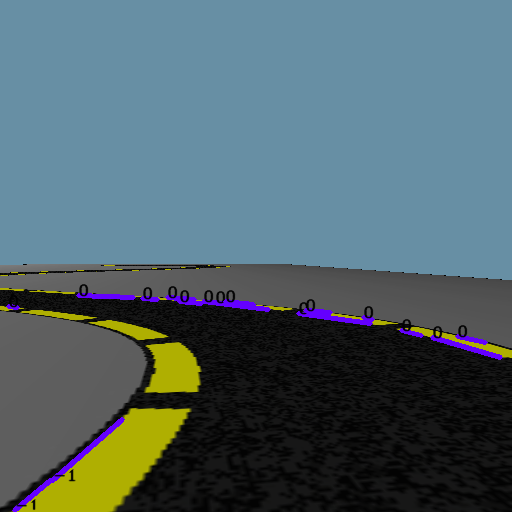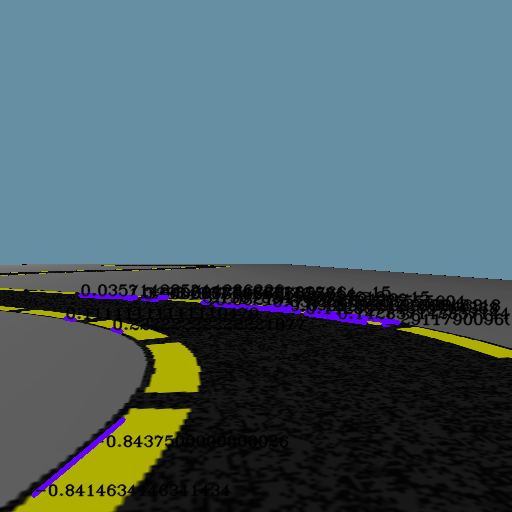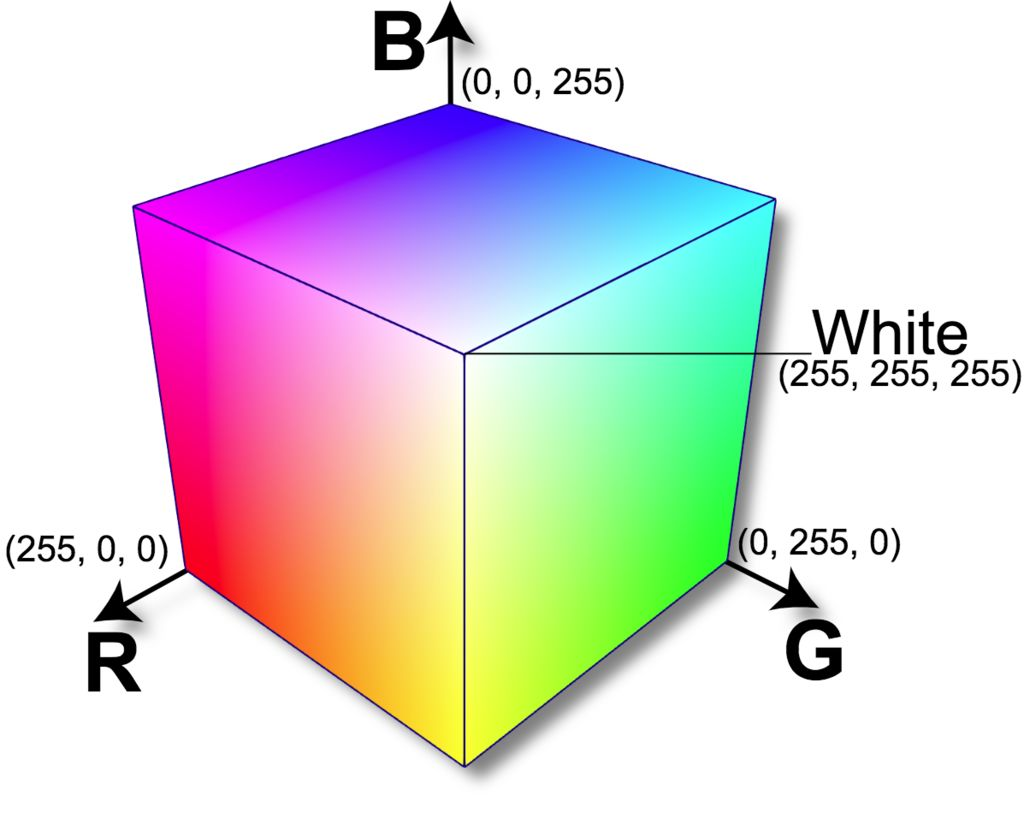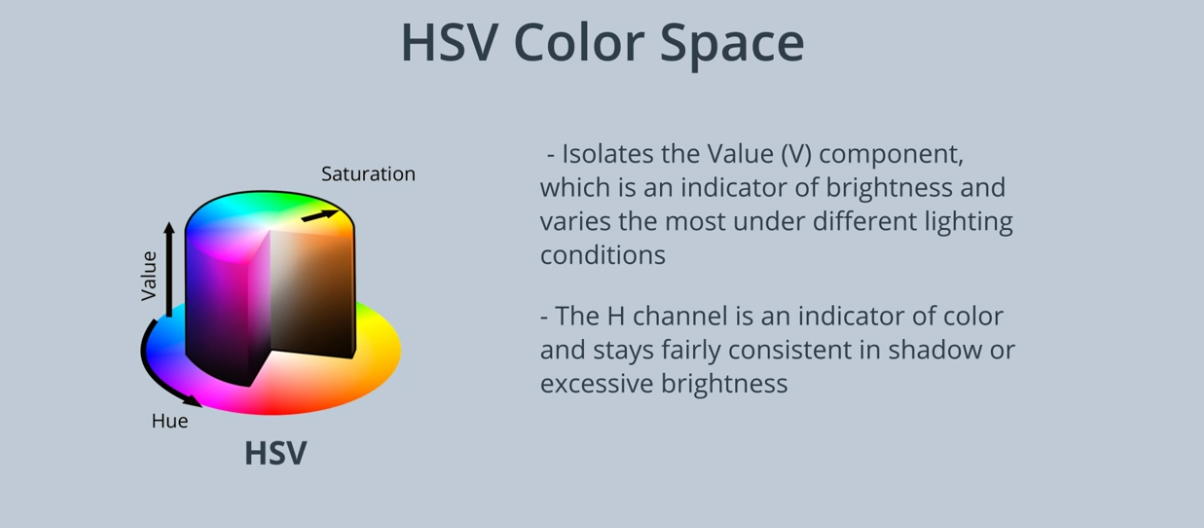# # Lane Tracking Robot

## # Algorithms

1. Get image

2. Store a copy of original image

3. Convert image to grayscale

4. Reduce noise with gaussian blur

5. Apply canny to get the sharp edges (canny works using differentiation of successive image pixels)

6. Find region of interest in the image in terms of a mask

7. AND the mask image with canny image to get only the lane portion

8. Apply HoughLineP with some filters to the resulting image to get the lines present in the image

9. Iterate through the lines to find the slope and y-intercept of each line'

10. Create two array of slope and y-intercept and insert the respective iteraed values based

on whether the slope is positive or negative to separate left and right lanes

11. Calculate the average of the two arrays to get the avergae slope and y-intercept of

left and right side of the lane

12. Make two lines for the lane using the average slope and y-intercept and using y1 as inage height and y2 as some value times y1

13. View the lines in the image

## # Can not compare only slope

### # Slope from own function

``````x1,y1,x2,y2 =line.reshape(4)
slope = (y2-y1)/(x2-x1)
intercept = y2 - slope * x2
``````
1
2
3### # Slope from built-in function

``````x1,y1,x2,y2 =line.reshape(4)
x_coords = (x1,x2)
y_coords = (y1,y2)
A = vstack([x_coords,ones(len(x_coords))]).T
slope, intercept = lstsq(A, ((y_coords)), rcond=None)
``````
1
2
3
4
5## # Color Masking using RGB color space and HSV Color Space

Color Masking is the process of detection of ceratin color in the image.

### # RGB Color Space### # HSV (Hue Saturation and Value) Color Space

This separate color and the brightness in the image as the separate component.They are highly useful for the color detection with various shadow and brightness

Unlike RGB which is defined in relation to primary colors, HSV is defined in a way that is similar to how humans perceive color.

The HSV color space represents colors using three values

1. Hue : This channel encodes color color information. Hue can be thought of an angle where 0 degree corresponds to the red color, 120 degrees corresponds to the green color, and 240 degrees corresponds to the blue color.

2. Saturation : This channel encodes the intensity/purity of color. For example, pink is less saturated than red.

3. Value : This channel encodes the brightness of color. Shading and gloss components of an image appear in this channel.